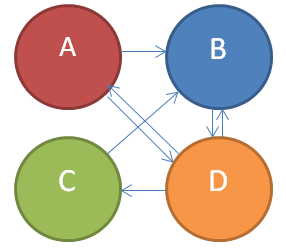## Saturday, February 9, 2013

### PageRank explained

Today, I thought I'd post about something near and dear to my heart: math.  When I was a senior at BYU (insert obligatory boos) studying numerical analysis, for one of my classes I wrote a paper about the PageRank algorithm.  Seeing as this is a web analytics class and that a big part of web analytics these days is search engine optimization, I thought I’d revisit the topic.  This time, though, I’ll do it in a way that is a lot simpler, involves less mathematical proofs, and I hope is less boring.
For those of you that don’t know what PageRank is, before Google adopted the “whoever gives the most money to Google wins” algorithm, Larry Page and Sergey Brin from Stanford developed a way to in essence let the internet itself determine the relative importance of the pages that it contains.  In the algorithm each member (page) of groups of hyperlinked documents (aka the internet) is assigned a weight based on the number of hyperlinks to it from other pages.  So, a page with a lot of links to it has a higher rank than a page with only a few links to it.

## How it worksSuppose we have an internet with 4 pages: A, B, C, and D with links to each other as illustrated below.  In this case, A has one link to it from D; B has a link to it from A, C, and D; C has one link to it from D; and D has 2 links to it, one from A and one from B.  Every time a page links to another page, it transfers a portion of its “rank” to the page that it links to.  So, D has a link to A, B, and C, so it transfers a third of its rank to A, a third to B, and a third to C.

So in our example, the ranks of each page are represented by the following equations:

Or, using matrix notation, it is the solution to the system of equations below.

Those of you who are mathematicians will notice that the PageRank values are an eigenvector of the matrix of link weights.  In our case, we want the one where the sum of all the ranks is 1.  So, for our model A=0.13, B=0.33, C=0.13, and D=0.4.
So, what exactly does this rank mean?  One interpretation is that that it is the probability that after following links for a long time you’ll end up on that particular page (If you try this on the real internet, you’ll likely either end up looking at Wikipedia or porn).
This is a simplified version of PageRank.  The actual algorithm is a bit different to take into account that not all pages have outbound links, people don’t just follow links all day when they surf the internet, etc., but this is essentially how it works.

## What it means for your site

Also, take note of where links to your page are coming from.  Remember that when a page links to yours, it transfers a portion of its PageRank to it.  A link from a big, important site is worth a lot more than a bunch of links from small, obscure sites.
1. http://en.wikipedia.org/wiki/Pagerank

1.2.3.